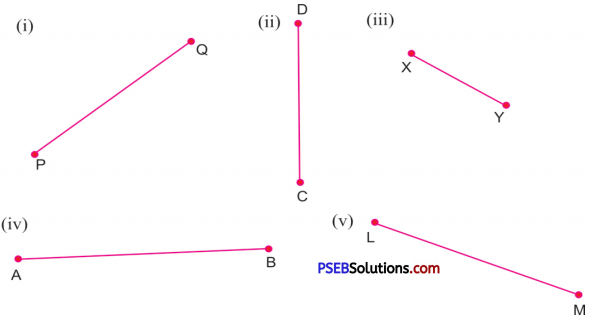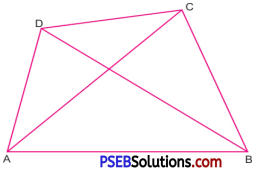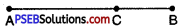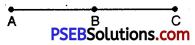# PSEB 6th Class Maths Solutions Chapter 9 Understanding Elementary Shapes Ex 9.1

Punjab State Board PSEB 6th Class Maths Book Solutions Chapter 9 Understanding Elementary Shapes Ex 9.1 Textbook Exercise Questions and Answers.

## PSEB Solutions for Class 6 Maths Chapter 9 Understanding Elementary Shapes Ex 9.1

1. Measure the line segments using a ruler and a divider:Solution:
(i) PQ = 4.4 cm
(ii) CD = 3.6 cm
(iii) XY = 2.5 cm
(iv) AB = 5.8 cm
(v) LM = 5 cm.2. Compare the line segments in the figure and fill in the blanks:Question (i)
AB _ AB
Solution:
AB = AB

Question (ii)
CD _ AC
Solution:
CD < AC Question (iii) AC _ AD Solution: AC > AD

Question (iv)
BC _ AC
Solution:
BC < AC Question (v) BD _ CD. Solution: BD > CD.

3. Draw any line segment AB. Take any point C between A and B. Measure the lengths of AB, BC and AC. Is AB = AC + CB?
Solution:
If A, B, C are any three points on a line such that AC + CB = AB, then we are sure that C lies between A and B.On measuring the lengths of AB, BC and AC, we get
AB = 6 cm, AC = 4 cm, CB = 2 cm
Now, AC + CB = 4 cm + 2 cm = 6 cm
Hence, AB = AC + CB.4. Draw a line segment AB = 5 cm and AC = 9 cm in such a way that points A, B, C are collinear. What is the length of BC?
Solution:AB = 5 cm and AC = 9 cm
Since, A, B and C are collinear
∴ AB + BC = AC
⇒ 5 cm + BC = 9 cm
⇒ BC = 9 cm – 5 cm
= 4 cm.
Hence, Length of BC = 4 cm